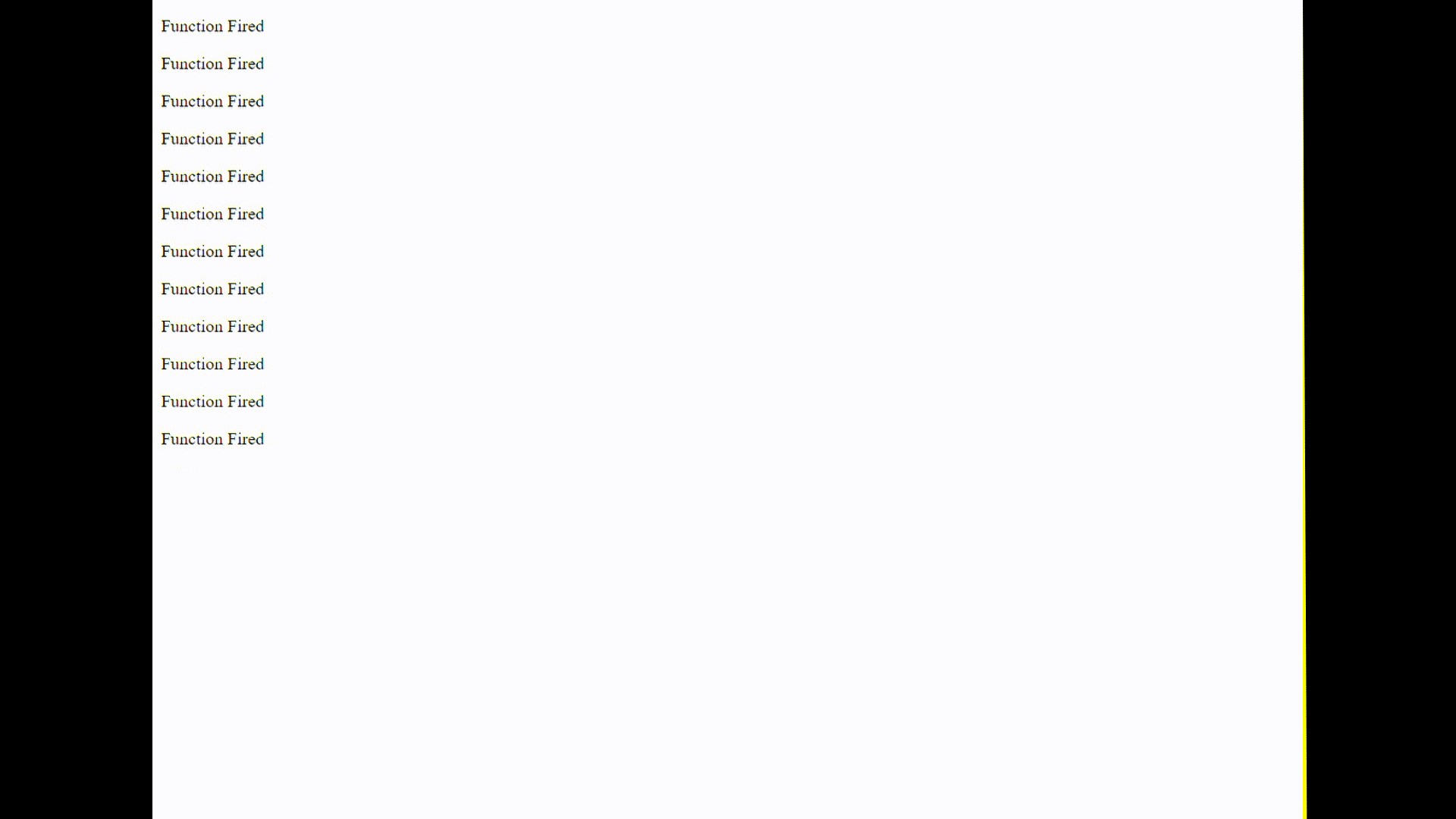Related Articles

# How to Change the Time Interval of setinterval() Method at RunTime using JavaScript ?

• Last Updated : 21 Jun, 2021

In this article, we are going to learn how to change the setInterval() time after running one element. The setInterval() method reads the timing once and invokes the function at regular intervals. There are two methods to solve this problem which are discussed below:
Method 1: Using clearInterval() method: The setInterval() method returns a numeric ID. This ID can be passed to clearInterval() method to clear/stop the setInterval timer. In this technique, we keep firing clearInterval() after each setInterval() to stop the previous setInterval() and initialize setInterval() with a new counter.
Example:

## html

 ```<``html``>``    ` `<``head``>``    ``<``title``>``        ``How to Change the Time Interval of``        ``setinterval() Method after Running``        ``one Element using JavaScript ?``    ````` `<``body``>``    ``<``div` `id``=``"message"``>``    ` `    ``<``script``>` `        ``// Output message``        ``var msg = document.getElementById("message");` `        ``var t = 200; // Timer``        ` `        ``// Stores the setInterval ID used by``        ``// clearInterval to stop the timer``        ``var interval;` `        ``f1();` `        ``// Function that changes the timer``        ``function changeTimer(){``            ``t = t * 1.2;``        ``}` `        ``// Function that run at irregular intervals``        ``function f1() {``            ` `            ``// Clears the previous setInterval timer``            ``clearInterval(interval);``            ``msg.innerHTML += "``<``p``>Function Fired``";``            ``changeTimer();``            ``interval = setInterval(f1, t);``        ``}``    ````` ``

Output:Method 2: Using setTimeout() method: This technique is easier and simpler than the previous one. The setTimout() method fires a function after a certain period. The timer is the time after which we want to run our function. In this technique, after performing the desired task, we change the value of the timer and call setTimeout() again. Now, since the value of timer changes, each function call is invoked at different intervals.
Example:

## html

 ```<``html``>``    ` `<``head``>``    ``<``title``>``        ``How to Change the Time Interval of``        ``setinterval() Method after Running``        ``one Element using JavaScript ?``    ````` `<``body``>``    ``<``div` `id``=``"message"``>``    ``<``script``>` `        ``// To display output``        ``var msg = document.getElementById("message");` `        ``// Timer``        ``var t = 200;` `        ``f1();``        ` `        ``// Function that changes the timer``        ``function changeTimer(){``            ``t = t * 1.2;``        ``}``        ` `        ``// Function to run at irregular intervals``        ``function f1() {``            ``msg.innerHTML += "``<``p``>Function Fired``";``            ``changeTimer();``            ``setTimeout(f1, t);``        ``}``    ````` ``

Output:My Personal Notes arrow_drop_up